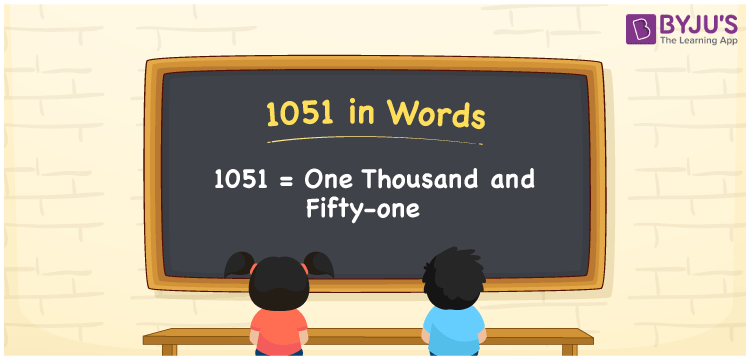# 1051 in Words

We can write 1051 in words as One thousand and fifty-one. Here, One thousand and fifty-one is called the number name of 1051. If you paid Rs. 1051 for preparing a particular dish in the hotel, you could say, “I paid One thousand and fifty-one rupees to prepare a special dish”. Let’s learn how to write the cardinal number in words using a place value chart.

 1051 in words One thousand and fifty-one One thousand and fifty-one in Numbers 1051

## 1051 in English Words

We generally write numbers in words using the English alphabet. So, we spell 1051 in English as “One thousand and fifty-one”.## How to Write 1051 in Words?

In this section, you will learn how to write the number 1051 in words using a four-column place value chart. This can be done as given below.

 Thousands Hundreds Tens Ones 1 0 5 1

Here, ones = 1, tens = 5, hundreds = 0, thousands = 1

These digits can be written as per the place values defined in the above table as:

1 × Thousand + 0 × Hundred + 5 × Ten + 1 × One

= 1 × 1000 + 0 × 100 + 5 × 10 + 1 × 1

= 1000 + 50 + 1

= 1000 + 51

= One thousand + Fifty-one

= One thousand and fifty-one

Therefore, 1051 in words = One thousand and fifty-one.

As we know, 1051 is a natural number that precedes 1052 and succeeds 1050.

1051 in words – One thousand and fifty-one

Is 1051 an even number? – No

Is 1051 an odd number? – Yes

Is 1051 a perfect square number? – No

Is 1051 a perfect cube number? – No

Is 1051 a prime number? – Yes

Is 1051 a composite number? – No

## Frequently Asked Questions on 1051 in Words

Q1

### How do you say 1051 in words?

You can spell the number 1051 in English words as One thousand and fifty-one.
Q2

### How to write out 1051 in word form?

The word form of the number 1051 is One thousand and fifty-one.
Q3

### Write an amount of Rs. 1051 in words.

We can write an amount of Rs. 1051 in words as “One thousand and fifty-one rupees”.